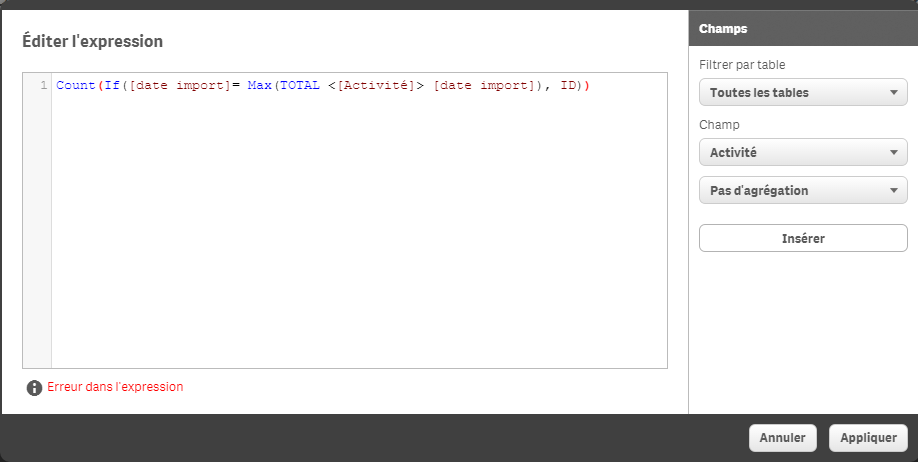# New to Qlik Sense

If you’re new to Qlik Sense, start with this Discussion Board and get up-to-speed quickly.

Announcements
Action-Packed Learning Awaits! QlikWorld 2023. April 17 - 20 in Las Vegas: REGISTER NOW
cancel
Showing results for
Did you mean:Partner - Creator III

## max() function for charts

Dear Community,

I'm tring to use the max() function combinated with count().

max([date import]) : gives me the date

Count(ID): gives me the number of ID

And what I want is to count only the ID when [date import] is max. So I tried different things:

Count(if( [date import]= max([date import]), ID))

Count({<max([date import])>} ID)

... It didn't work...

I'm sure it's possible ! thanks for your help

Best regards

1 Solution

Accepted SolutionsMVP

Try this:

=Count({<[date import] = {"\$(=Date(Max([date import]), 'DateFormatHere'))"}>}ID)

18 RepliesMVP

Try this:

=Count({<[date import] = {"\$(=Date(Max([date import]), 'DateFormatHere'))"}>}ID)MVP

Or may be this:

Count(If([date import]= Max(TOTAL [date import]), ID))Partner - Creator III
Author

That was very fast Sunny. It worked perfectly! Many thanks !MVP

AwesomeBest,

SunnyPartner - Creator III
Author

Hi Sunny,

Both fonction work. But I tried to use it in an other chart and I get the following problem. As you can see in the first chart I was counting the ID for each Station. For each station you can have several dates ([date import]). And what I did priviously because I wasn't able to use max() is to count all the ID and divided it by the number of distinct [date import]: Count(ID)/(count(distinct([date import]))).

So I tried both answers you send me because I'm more interested by the number of ID at max([date import]) for each Station instead of my average calculation. But it seems that Qlik will first look for the max([date import]) and then look for the station. The result is on the second picture where you can see that there is only one point.

So how to tell Qlik that for each station I want to look for the max([date import]) and then count the ID. instead of looking for the max([date import]) count the ID and then look for the station?MVP

See if this works:

Count(If([date import]= Max(TOTAL <Station> [date import]), ID))Partner - Creator III
Author

Qlik tells me there is an error in the expression. I tried a few changes but no results so far. I will dig a little more and see what I can get. Anyway I don't want to bother you for this, you have been more than helpfull!

Many thanks again Sunny !MVP

‌Can you share a screenshot of the error you are seeing when you use the expression I gave you? Include the expression and the error message both.

Best,

SunnyPartner - Creator III
AuthorStation is called "Activité" in my data base. Tool is in frenchTags
Community Browser